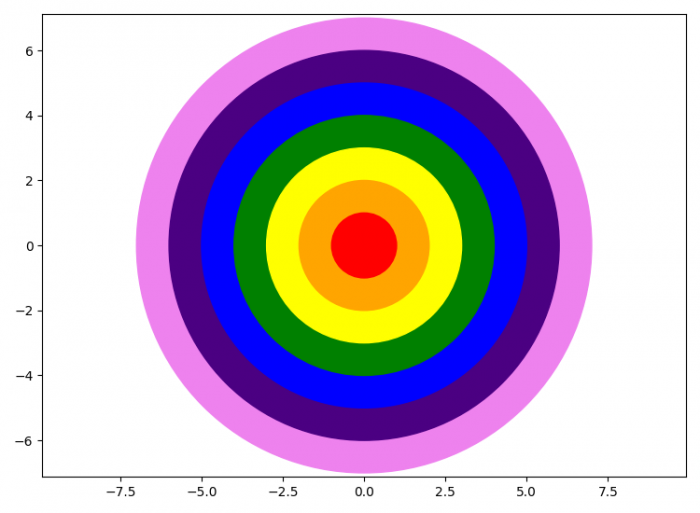# How to plot a rainbow cricle in matplotlib?

To plot a rainbow circles in Matplotlib, we can take the following steps −

• Set the figure size and adjust the padding between and around the subplots.
• Create a figure and a set of subplots.
• Set the X and Y axes scale.
• Make a list of rainbow colors.
• Create a true circle at (0, 0).
• Add a circle instance 'c' to the figure.
• To display the figure, use show() method.

## Example

import matplotlib.pyplot as plt

plt.rcParams["figure.figsize"] = [7.50, 5.50]
plt.rcParams["figure.autolayout"] = True

fig, ax = plt.subplots()
plt.axis("equal")

ax.set(xlim=(-10, 10), ylim=(-10, 10))

for i in range(0, 7):
rainbow = ['violet', 'indigo', 'blue', 'green', 'yellow', 'orange', 'red']
c = plt.Circle((0, 0), 7-i, fill=True, color=rainbow[i])
plt.show()